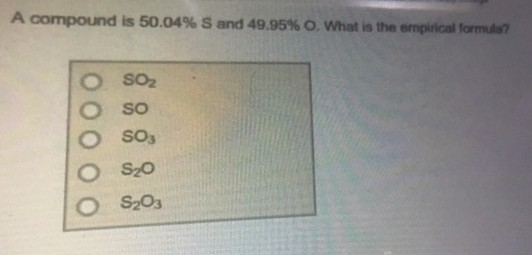# Problem: A compound is 50.04% S and 49.95% O. What is the empirical formula? a. SO2 b. SOc. SO3 d. S2Oe. S2O3

###### FREE Expert Solution
79% (269 ratings)###### Problem Details

A compound is 50.04% S and 49.95% O. What is the empirical formula?

a. SO2

b. SO

c. SO3

d. S2O

e. S2O3